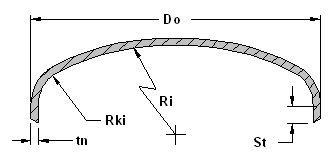Pressure Vessel Flanged & Dish Head Design Tool Calculator

The following are equations and a calculator to determine the wall thinkness and working pressure of an ellipsoidal head with t/L greater than or equal to 0.002.

The require thickness of a dished semiellipsoidal head in which one-half of the "minor axis" (inside head minus the skirt) is equal to one-fourth of the inside diameter of the head skirt.

The following equations apply:

ThicknessPressureDimensions: Ri [in] - inside crown radius Rki [in] - inside knuckle radius tn [in] - thickness before forming tf [in] - thickness after forming Ca [in] - corrosion allowance 1.800 St [in] - straight skirt length S [psi] - allowable stress E - head longitudinal efficiency P [psi] - internal pressure Variables: nt [in] = Ri/Rki M = Ro [in] = Required Thickness: Tr [in] = Thickness Check: Maximum Pressure: Po [psi] = Pressure Check:

Where:

Do = outside diameter

tn = nominal thickness before forming

tf = thickness after forming

Ca = corrosion allowance

St = skirt length (straight section)

S = maximum allowable stress value

E = joint efficiency for, or the efficiency of, appropriate joint in cylindrical or spherical shells, or the efficiency of ligaments between openings, whichever is less.
For welded vessels, use the efficiency specified in UW-12. For ligaments between openings, use the efficiency calculated by the rules given in UG-53.

P = internal design pressure

nt = tf - Ca = thickness with corrosion allowance removed

Ri/Rfi = Ri/Rki

M = 0.25(3 + sqrt(Ri/Rkii))

Ro = Ri + tn

Tr = Required design thickness

Po = maximum operating pressure

References: ASME Boiler and Pressure Vessel Code

Contributed by:

Harsh Murugesan
Pune, Maharashtra IndiaMembership Register | LoginHomeEngineering Book StoreEngineering ForumExcel App. DownloadsOnline Books & ManualsEngineering NewsEngineering VideosEngineering CalculatorsEngineering ToolboxGD&T Training Geometric Dimensioning TolerancingDFM DFA TrainingTraining Online EngineeringAdvertising CenterCopyright Notice